# New Performance Index “Attractiveness Factor” for Evaluating Websites via Obtaining Transition of Users’ Interests

## Abstract

The studies of browsing behavior have gained increasing attention in web analysis for providing better service. Most of the conventional approaches focus on simple indices such as average dwell time and conversion rate. These indices make similar evaluations to websites even if their features are significantly different. Moreover, such statistical indices are not sensitive to the dynamics of users’ interests. In this paper, we propose a new framework for measuring a website’s attractiveness that takes into account both the distribution and dynamics of users’ interests. Within the framework, we define a new index for the website, called Attractiveness Factor, which evaluates the degree of users’ attention. It consists of three procedures: First, we capture the transition of users’ interests during browsing by solving a nonnegative matrix factorization and constrained network flow problems. To accommodate multiple types of interests of a user, we applied a soft clustering as opposed to a hard clustering to model attributes of users and websites. Second, for each website, the feature of each cluster is obtained by fitting the dwell time distribution with Weibull distribution. Finally, we calculate Attractiveness Factor of a website by applying the results of clustering and fitting. Attractiveness Factor depends on the distribution of the dwell time of users interested in the website, which reflects the change of interest of users. Numerical experiments with real web access data of Yahoo Japan News are conducted by solving extremely large-scale optimization problems. They show that Attractiveness Factor captures more exceptional information about browsing behavior more effectively than well-used indices. Attractive factors give low ratings to category pages; however, it can assign high ratings to websites that attract many people, such as hot topic news about the 2018 FIFA World Cup, Japan’s new imperial era’ REIWA,’ and North Korea—the United States Hanoi Summit. Moreover, we demonstrate that Attractiveness Factor can detect the tendency of users’ attention to each website at a given time interval of the day.

## Introduction

Browsing behavior has been actively researched in the field of web analysis. One of the challenging problems is defining a critical metric that accurately measures the website’s performance. Some services analyze browsing behavior using various indices such as average dwell time on a website, the number of visitors, and exit rate, which are easily calculated using the access log. In this paper, we introduce a new index, called “Attractiveness Factor,” which enables us to evaluate websites via obtaining the transition of users’ interests. Users have various interests that dynamically change over time; thus, we focus on two essential features to capture them; dynamics and distribution.

Dynamics represents users’ changing interests for webpages depending on situations and purposes. Most existing works have utilized static information such as visited webpages and dwell time on the webpage to obtain users’ individual information which does not change among webpages. It can be acquired by applying machine learning techniques such as k-means [11, 25] or c-means [7, 21] or other clustering methods. However, assuming daily use, our interest in each webpage is always changing. Therefore, it is reasonable that users’ access pattern reflects such dynamics of users’ interests. We can obtain these kinds of dynamics by utilizing access sequences, which are the successive access logs. Based on access sequences, we apply the constrained network flow problem to obtain dynamic information. It enables us to interpret users’ occasional access motivations from access sequences.

On the other hand, distribution plays a vital role to understand the whole tendency of behavior on each webpage. Most conventional approaches  for web usage mining focus on simple indices such as average dwell time and conversion rate. They ignore the dwell time distribution of websites; hence, these indices make similar evaluations for different websites. The dwell time indicates how long a user spends on a website, and its distribution enables us to gather more information than simple indices. To take advantage of the dwell time distribution, we apply Weibull distribution, which is widely utilized for analyzing the system failure in reliability engineering. Previously, Liu et al.  made an analogy between the failure rate of products and users’ dwell time on a website. The shape parameter of Weibull distribution, which fits dwell time distribution, yields the degree of users’ interests. The study mentioned above attempted to fit dwell time distribution using only single Weibull distribution. We expand on this study and apply the mixture Weibull distribution because users generally behave differently depending on their interests and the purpose of browsing. To obtain the mixture Weibull distribution, we identify users’ membership to each cluster, which is detailed in Sect. 5.

Based on the approach mentioned above, we propose a new framework that measures a website’s attractiveness that considers both the distribution and dynamics of the users’ interests. Our framework consists of three procedures. First, we capture the transition of users’ interests during browsing by solving a nonnegative matrix factorization and constrained network flow problems. To account for the various interests of a user, we adopt soft clustering as opposed to hard clustering to model the attributes of users and websites. Second, for each website, the feature of each cluster is obtained by fitting the dwell time distribution with Weibull distribution, which is well known as a useful tool for analyzing the system failure in reliability engineering. Finally, we calculated the Attractiveness Factor of a website by applying the results of clustering and fitting with Weibull distribution. Attractiveness Factor depends on the distribution of dwell time of users interested in the website, which reflects the changing interest of users. The motivation for proposing this index is entirely different from those of existing indices in previous works [15, 19, 24, 26].

Numerical experiments are conducted by solving extremely large-scale optimization problems. We developed three datasets from the real access data of Yahoo Japan News for the morning, for the daytime, and night. Each of these datasets contains access data for an hour during the periods mentioned above of the day. Numerical experiments show that the Attractiveness Factor successfully reflects the change of users’ interests, which is hardly achieved in well-used indices. In summary, our main contributions are as follows:

• We propose a new soft clustering method to obtain users’ dynamics for each website by utilizing nonnegative matrix factorization and constrained network flow problems.

• We propose a new performance index, called Attractiveness Factor, for evaluating a website by fitting users’ dwell time distribution with a mixture Weibull distribution. We can understand how the website retains the users’ attention.

• We conduct numerical experiments to demonstrate the potential of Attractiveness Factor and the advantages of our clustering method by applying extremely large-scale real access data of Yahoo Japan News.

Our proposed framework is summarized in Fig. 1. The remainder of this manuscript is organized as follows. We briefly discuss the related works in these field in Sect. 2 and explain the background of this research in Sect. 3. In Sect. 4, the proposed index “Attractiveness Factor” is defined, and in Sect. 5, the novel method of clustering users’ browsing behavior is introduced. We describe the appropriate method in Sect. 6, and we summarize the results of the numerical experiments in Sect. 7.Fig. 1

## Related Works

### Web Structure Mining

Web structure mining analyzes properties of a graph made from the websites and their hyperlinks. These researches are mainly inspired by the study of social networks and citation analysis .

HITS  is one of the most well-known mathematical theory-based indices using the web structure. This algorithm is based on a hypothesis that most websites belong to either hubs or authorities. Authorities have much information about a particular topic. On the contrary, hubs collect hyperlinks to authorities. As a result, there is a bipartite subgraph between authorities and hubs in the web graph. HITS identifies authorities and hubs for a given topic by calculating the authority score and the hub score from the web graph.

Another example of a popular method for evaluation is PageRank , which employs a discrete-time Markov process as the model. Liu et al.  attacked the weakness of PageRank and introduced the Browse rank, which can be calculated from a directed graph representing transitions between websites in the users’ web browsing history. Lagun and Lalmas  focused on more details of a website and defined Viewport time, which attempted to determine which part of the website a user stays on to predict user engagement. Bounce rate , which is the rate of users who quit browsing without transitioning, can be calculated using Google Analytics. Such analytics tools enable us to obtain well-used indices such as the average dwell time on webpage, exit rate, and number of visitors. Budylin et al.  focused on the problem of sensitivity improvement of well-used indices. They proposed a new transformation of a ratio criterion to the average value that created an opportunity to directly use a wide range of sensitivity improvement techniques designed for the user level that make A/B tests more efficient. We summarize the difference from indices mentioned above to the proposed index in Table 1: It indicates that the focus of existing works is entirely different from that of our work.

### Web Usage Mining

There is another stream for evaluating websites; web usage mining focuses on users’ behavior to detect usage patterns of websites. There are various data for users’ behavior, such as access sequence, dwell time, and click behavior.

Many researchers have interests in users’ dwell time on each website. Liu et al.  found the analogy between dwell on website and object defects for the first time. They regarded the abandoning a browsed page as a failure of the system and fit the dwell time distribution with Weibull distribution. Kim et al.  attempted to demonstrate the dwell time by fitting Gamma distribution and showed the relationship between users’ satisfaction and their dwell times. In contrast, some researches did not consider certain distributions. These approaches are referred to as nonparametric models. Vasiloudis et al.  predicted users’ dwell time on the website by the gradient-boosted tree, and Barbieri et al.  introduced the knowledge of survival analysis and addressed the relationship between the feature of ads and dwell time. Wang et al.  attempted to predict users’ dwell time at specific points using factorization machines. Nikolaev et al.  proposed decomposing the dwell time distribution into two distributions without considering specific distributions, which enables reactions of high sensitivity to A/B tests .

Other many studies also have attempted to tackle user clustering for understanding user’s behavior and recommending other websites. The most basic method of clustering is k-means clustering [11, 25]; however, this method is not appropriate for our task because each user belongs to only one cluster. Many other clustering methods that allow users to belong to multiple clusters have been proposed. Most of them have attempted to apply c-means clustering [7, 21], Gaussian mixture model. Some other researches attempted to apply the CARD algorithm . Gopalakrishnan and Sengottvelan  attempted to perform clustering using a naive Bayesian classifier. Khusumanegara et al.  applied hierarchical agglomerative clustering. Bhavithra and Saradha  proposed a new clustering method based on the k-NN approach as preprocessing for a recommendation. Recently, some research approached this problem using neural networks to predict each user’s movement, such as . Lu et al.  first focused on changing the user’s interest between before visiting the website and after it. However, in the study mentioned above, satisfaction is gathered through a questionnaire, which involves considerable cost.

## Background

### Weibull Distribution

Weibull distribution has been used for analyzing the object defect, and a previous work  drew an analogy between abandoning a website and system failure in reliability analysis. The probability density function of Weibull distribution is as follows:

\begin{aligned} f(t) = {\left\{ \begin{array}{ll} \frac{m}{\eta } \left( \frac{t}{\eta } \right) ^{m-1}\exp \left\{ \left( -\frac{t}{\eta }\right) ^m \right\} &{}( t > 0) \\ 0 &{}{\mathrm{(otherwise)}} \end{array}\right. } \end{aligned}

where the parameters m and $$\eta$$ are called the shape parameter and the scale parameter, respectively. Figure 2 shows how the shape parameter m affects the distribution.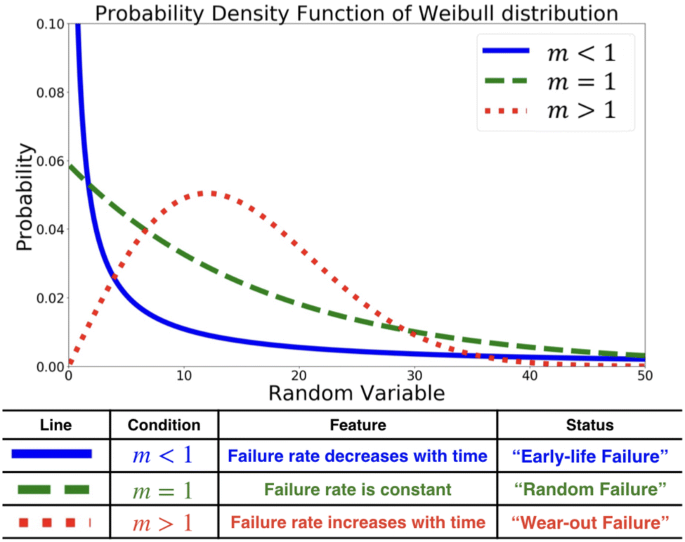Fig. 2

In terms of object defect, the distribution represents limited early failure if the shape parameter m is greater than 1. Similarly, if users’ dwell time distribution on a website follows a single Weibull distribution whose m is greater than 1, then few people move away soon after opening the website; therefore, the website can be considered attractive.

### Pre-experiment of Utilizing Mixture Weibull Distribution

Previously,  attempted to fit only single Weibull distribution and most websites (98.5%) have m less than 1, which means that many people moved away from these websites soon after opening them. As mentioned in Sect. 3.1, the parameter m reflects the degree of users’ interests, which is represented as their access patterns. There are different access patterns in a website corresponding to factors such as interests and purpose. Therefore, a mixture Weibull distribution could be suitable for analyzing users’ dwell time distribution. To verify our hypothesis, we fitted single and double Weibull distribution with real access data of Yahoo Japan Bookstore on August 30, 2017. We compared the performance of fitting by calculating the Kullback–Leibler divergence, which measures how one probability distribution diverges from the other. In general, assuming p(x) and q(x) are continuous probability distributions, Kullback–Leibler divergence from p(x) to q(x) is defined as follows,

\begin{aligned} \text{KL}\left( p(x)\Vert q(x)\right) = \int _{-\infty }^{\infty } p(x)\log \frac{p(x)}{q(x)} {\rm d}x. \end{aligned}

We input users’ dwell time distribution as p(x) and the fitted Weibull distributions as q(x). The results of the experiment are shown in Fig. 3. The results show that the double Weibull distribution performs much better than single Weibull distribution. The double Weibull distribution comprises two Weibull distributions. The shape parameter of one Weibull distribution is greater than 1, and that of the other is less than 1. This implies that some clusters corresponding to the degrees of users’ interests and that our hypothesis may be correct.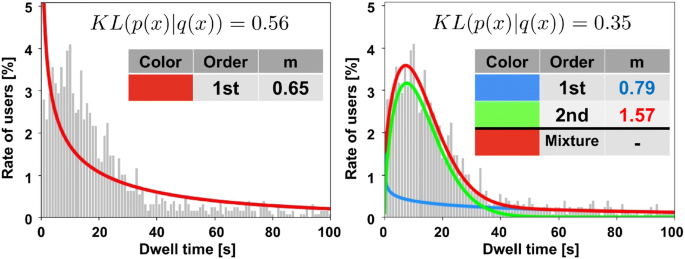Fig. 3

Based on the mentioned small experiment, we fit one to five mixture Weibull distribution to users’ dwell time distribution with large datasets. We picked up two days and utilized three datasets per day, which contains a 1-h real access log of Yahoo Japan News. In total, we utilized six datasets. We fit one to five mixture Weibull distribution with many websites and calculated Akaike information criteria (AIC). The average of AIC is summarized in Table 2.

Based on these experiments, our framework employs a mixture Weibull distribution to analyze users’ dwell time distribution.

### Motivation of Founding New Performance Index

To obtain the mixture Weibull distribution, we must identify the degree of users’ interests. We must pay attention to the fact that each person behaves differently on each website because the degree of each user’s interest is different on each website. Therefore, it is unfavorable to fix the users’ membership of each website. Most researches have not considered the dynamics of users’ interests. As mentioned in Sect. 1, considering the dynamics of users’ interests is essential to develop a key metric.

Therefore, we propose a new soft clustering method that considers the change in the user’s interest in each website. After applying the proposed soft clustering method, we fit users’ dwell time distribution of each cluster with a single Weibull distribution. Finally, we can accurately evaluate the website’s attractiveness using the proposed index, “Attractiveness Factor.” This index is defined using the shape parameter of Weibull distribution and the percentage of users for each cluster, which is defined in Sect. 4.

## Definition of Attractiveness Factor

In this section, we define the proposed index “Attractiveness Factor” (AF) which focuses on users’ dwell time distribution. If one website has much attraction, we assume the two following reasonable conditions:

1. (1)

The proportion of people who are interested in the website is plentiful.

2. (2)

Many people tend to stay on the website for an extended amount of time.

We rephrase these conditions in terms of the Weibull distribution. When Weibull distribution is applied to the manufacturing industry, if the shape parameter m of the fitted Weibull distribution is greater than 1, then the failure is small in the early stage, and the breakdown increases after an extended amount of time, then it is regarded as a product with constant durability. After fitting Weibull distribution with users’ dwell time distribution, if the website has attractiveness, the parameters of Weibull distribution satisfy the following conditions:

$$(1)^{'}$$:

Many people belong to a cluster whose shape parameter of Weibull distribution is greater than 1.

$$(2)^{'}$$:

The shape parameter is sufficiently larger than 1.

Under these assumptions, we define AF of the website s denoted by AF(s) as follows:

\begin{aligned} AF(s) := \mathop{\mathop{\sum}\limits_{k = 1}}\limits_{m^s_k > 1}^{K} \frac{N^s_k}{N^s}{m^s_k} \end{aligned}

K : Number of clusters, $$N^s$$ : Number of users visiting a website s, $$N^s_k$$ : Summation of the rate of members belonging to the cluster k over users visiting the website s, $$m^s_k$$ : Shape parameter of Weibull distribution generated from the cluster k of the website s.

We sum over k only with $$m_k^s > 1$$ because $$m_k^s \le 1$$ indicates that the users have less interest in that website, according to the characteristics of Weibull distribution (summarized in Fig. 2). In addition, the parameter $$\eta$$ of Weibull distribution is not considered by AF, which is related to the average dwell time. This is because the meaning of “long stay” is different between websites corresponding to the volume and topic of the website.

## Browsing Behavior Clustering

In this section, we propose our soft clustering algorithm, which considers users’ changeable interests during browsing. Hard clustering is not appropriate because users may have several interests when visiting the website. Moreover, many researchers tackled problems under the constraint that the memberships of clusters are fixed at any given time. However, considering daily use, users’ interests can transition depending on factors such as interest and time. Therefore, we propose a soft clustering method that can transition the membership of clusters for each website as a network flow. First, we explain the data format and the preprocessing algorithm in Sect. 5.1. In Sect. 5.2, we obtain the overall users’ interests and the features of each website by performing nonnegative matrix factorization (NMF). Next, in Sect. 5.3, we describe the decomposition of the number of transitions into cluster transitions. Finally, the transition of each user’s interest during browsing is acquired as a network flow in Sect. 5.4. In Sects. 5.3 and 5.4, we solve constrained network flow problems formulated as linear optimization problems.

### Definition and Preprocess

We define sequence as the list of websites where one user browsed without leaving from the Internet; in other words, it is the successive access logs. Access data records that when and where the user visited and left. The data format is shown in Table 3. Each element of it shows the three websites (Last, Origin, and Destination ) which the user visited.

We introduce how to retrieve sequence from the access log. We extract the access log for each user to retrieve the access sequence as a preprocess. We can not get an access sequence by simply connecting access logs in chronological order on following such situations (Fig. 4)

• A user returns to a website that has already been opened.

• A user browses with opening multiple tabs.

Figure 4 illustrates the example of converting the access log shown in Table 3 to the access sequence; the number means the order of browsing with opening two tabs. We construct an access sequence by following steps,

• We construct a multi-directed graph with connecting two webpages if there are two webpages that Origin of the former log and Last of the latter log are identical in the access log.

• We repeat to obtain a path called access sequence from the root node to a leaf node and to remove edges used in the access sequence.

• We calculate a dwell time per each node on every access sequence.

After applying for the mentioned preprocess in Fig. 4, we obtain two access sequences [A, B, C, D] and [B, E, F, G].Fig. 4

### Picking Average Feature of Users and Websites

In the first step of our clustering method, overall users’ interests and websites’ features are selected by using nonnegative matrix factorization (NMF). NMF has been used in image processing , co-clustering , and sound analysis. NMF  aims to decompose a large-scale matrix into low-rank latent factor matrices with nonnegative constraints. The input data are each users’ dwell time on each website, and the output data are each user’s feature vector and each website’s feature vector. Let U and S be the set of users and set of websites, respectively, and the number of users and the number of websites are denoted by $$N := |U|, P := |S|$$. Further, let $$T \in {\mathbb {R}}^{N \times P}$$ be the input matrix for NMF, and each element $$T_{{\text{us}}}$$ is the dwell time on a website s by user u.

NMF is a type of optimization problem and can be formulated as follows:

\begin{aligned} \underset{W,H}{\mathrm{minimize}}&\quad \Vert T -WH \Vert _F^2 \\ {\mathrm{subject \ to}}&\quad W,H \ge 0 , \quad W \in {\mathbb {R}}^{N \times K} , \quad H \in {\mathbb {R}}^{K \times P}. \end{aligned}

There are some definitions of distances to run NMF, such as Euclidian distance and Itakura–Saito divergence. In this paper, we apply Euclidian distances.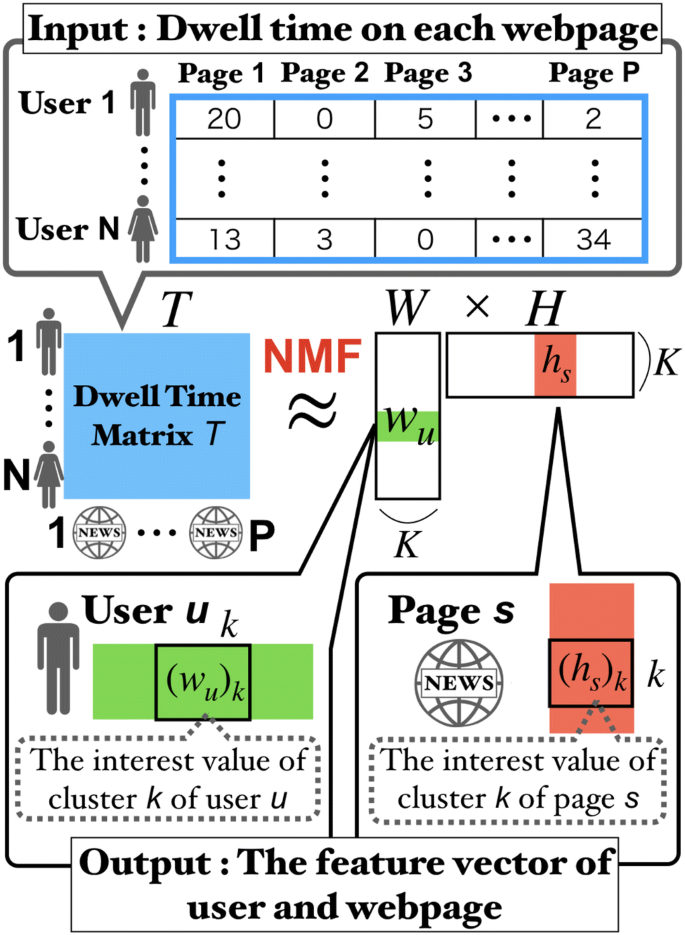Fig. 5

Using NMF, we decompose matrix T ($$N\times P$$ matrix) into two matrices, W ($$N\times K$$ matrix) and H ($$K\times P$$ matrix). Row u of W and column s of H represent the K features of user u and website s, respectively (see Fig. 5). In other words, the feature of each user and each website can be obtained as a K-dimensional vector. Based on the K-dimensional website’s feature vector, we make K clusters on a website. When a user visits the website, the user has a K-dimensional vector, which represents the membership for each cluster. Note that the feature vectors of each website and each user are standard features, and each user does not always have the same interests.

We must input K (the number of features) before performing NMF. We apply the results of previous studies , which suggests choosing K so that the magnitude of the cophenetic correlation coefficient begins to decline.

### Decomposing the Number of Transitions into Some Flows

In this section, we do not focus on each user, but rather the number of users that travel between clusters (shown in Fig. 6). To obtain the number of people traveling between clusters, we formulate and solve a linear optimization problem based on the output of NMF.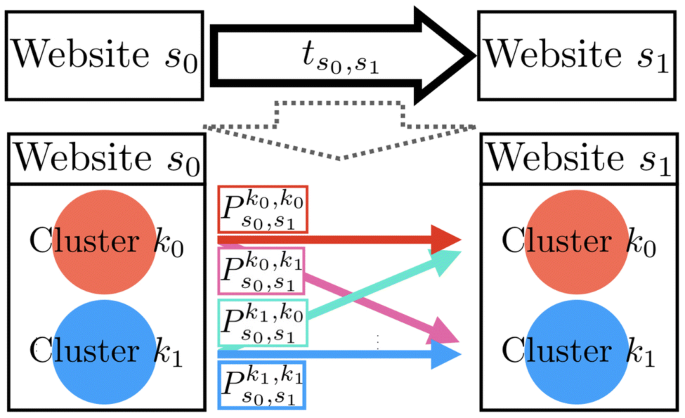Fig. 6

In the remainder of this paper, we use the following definitions:

Definitions

• U : Set of users

• $$N^{s}$$ : Number of users visiting a website s

• S : Set of websites

• $$S_u$$ : Set of websites which user u visited

• $$S_0$$ : S$$\cup$$$$\{{\mathrm{super \ source}}\}$$, $$S_1$$ : S$$\cup$$$$\{{\mathrm{super \ sink}}\}$$

• $$t_{s_0,s_1}$$ : Number of users traveling from website $$s_0$$ to $$s_1$$

• $$t_{s_1}$$ : Number of users visiting $$s_1$$

• $$\varvec{w}_u$$ : Feature vector of user u (output of NMF)

• $$\bar{\varvec{w}}_u$$ : Normalized vector of $$\varvec{w}_u$$

• $$\varvec{h}_s$$ : Feature vector of website s (the output of NMF)

• $$\bar{\varvec{h}}_s$$ : Normalized vector of $$\varvec{h}_s$$

Moreover, let $$P^{k_0,k_1}_{s_0,s_1}$$ be the number of people who move from cluster $$k_0$$ in website a $$s_0$$ to the cluster $$k_1$$ in website $$s_1$$. This is the objective variable of this part of the optimization problem, and note that this variable can yield a real continuous value. We regard people’s transition between websites as a network flow. The super source and the super sink are set, and every user comes from the super source and goes to the super sink. We describe certain constraints as follows:

(a) Each website has a feature vector after solving NMF. We attempt to bridge the gap between the proportion of clusters and to the feature vector, which is denoted by $$\varepsilon _1$$

\begin{aligned} \left| \frac{1}{t_{s_1}}\sum _{s_0 \in S_0} \sum _{k_0 = 1}^{K} {P^{k_0,k_1}_{s_0,s_1}} - (\bar{\varvec{h}}_{s_1})_{k_1} \right| \le \varepsilon _1 \ (\forall s_1,k_1). \end{aligned}
(1)

We show two examples, both of which satisfy constraint (1) in Fig. 7.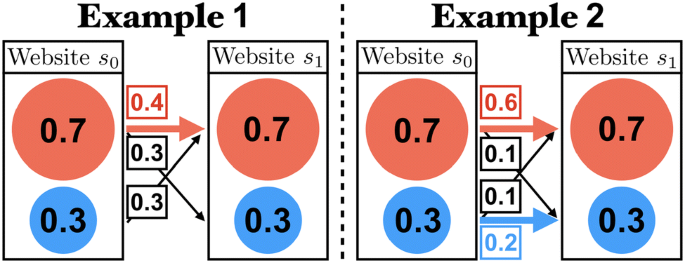Fig. 7

(b) When the user travels between two similar websites, the user tends to belong to same cluster in the two websites. In Fig. 7, some fewer users changed their cluster membership, in example 2 than in example 1; hence, the case in example 2 is more practical. The more significant two websites have the similarity, the more we make many users belong to the same cluster between two websites. The gap between the similarity and the rate of users belonging to the same cluster should be small, which is denoted by $$\varepsilon _2$$

\begin{aligned} \left| \frac{1}{t_{s_0,s_1}}\sum _{k_0 = 1}^{K} {P^{k_0,k_0}_{s_0,s_1}} -\rho _{s_0,s_1} \right| \le \varepsilon _2 \ (\forall s_0,s_1) \end{aligned}
(2)

We apply “cosine similarity” for measuring the similarity between website $$s_0$$ and website $$s_1$$ by applying the feature vector $$\varvec{h}$$ of the website as the output of NMF.

\begin{aligned} \rho _{s_0,s_1} = \frac{\varvec{h_{s_0}} \cdot \varvec{h_{s_1}}}{\Vert \varvec{h_{s_0}}\Vert \Vert \varvec{h_{s_1}}\Vert } \end{aligned}
(cosine similarity)

(c) If each website has K clusters, there are $${K^2}$$ ways from one website to another website. The number of users who transition between them is equal to the sum of the number of users who pass each way (shown in Fig. 6).

\begin{aligned} \sum _{k_0, k_1 = 1}^{K}{P^{k_0,k_1}_{s_0,s_1}} = t_{s_0,s_1} \ (\forall s_0,s_1) \end{aligned}
(3)

(d) For each cluster of each website, the number of people who came and left is the same (flow conservation).

\begin{aligned} \sum _{s_0 \in S_0} \sum _{k_0 = 1}^{K} P_{s_0,s_1}^{k_0,k_1} = \sum _{s_2 \in S_1} \sum _{k_2 = 1}^{K} P_{s_1,s_2}^{k_1,k_2} \ (\forall s_1,k_1) \end{aligned}
(4)

Constraints (1) and (2) are difficult to implement; hence, this optimization problem aims to make these gaps as small as possible. In other words, the minimization of the summation of these parameters is the objective function.

\begin{aligned} \underset{\varepsilon _1,\varepsilon _2}{\mathrm{Minimize}}&\quad \varepsilon _1 + \varepsilon _2 \\ {\mathrm{subject \ to}}&\quad {\mathrm{(a), (b), (c) \ and \ (d)}}\\&P^{k_0,k_1}_{s_0,s_1} \ge 0 \ (\forall k_0,k_1,s_0,s_1) , \quad \varepsilon _1, \varepsilon _2 \ge 0. \end{aligned}

As a result of solving this problem, we obtain $$P^{k_0,k_1}_{s_0,s_1}$$ as the number of users who transition from one cluster in one website to a cluster on another website.

### Obtaining the Changeable Membership as Network Flow

In Sect. 5.2, we acquired the feature vector of each user by performing NMF (see in Fig. 5), and we obtained the number of user transitions between clusters in Sect. 5.3. Based on this information, we determined how users navigating between websites change their interests by solving the constrained network flow problem.

#### Obtaining Reasonable Transition of Users’ Interests

First, we obtain a reasonable persons’ interest transition. Let $$(X_u^{s_0,s_1})_{k_0,k_1}$$ be the rate of transitioning from website $$s_0$$, belonging to cluster $$k_0$$, to website $$s_1$$, belonging to cluster $$k_1$$, of the user u. We can obtain each user’s membership of each website by utilizing the matrix X, which is calculated as follows.

\begin{aligned} x_{u_{k_1}}^{s_1} := \sum _{k_0 = 1}^{K}(X_u^{s_0,s_1})_{k_0,k_1}. \end{aligned}

We call this vector the dwell vector. Figure 8 shows the example in a case where $$K=3$$, in which the user browses websites while changing interests, and each color represents each cluster.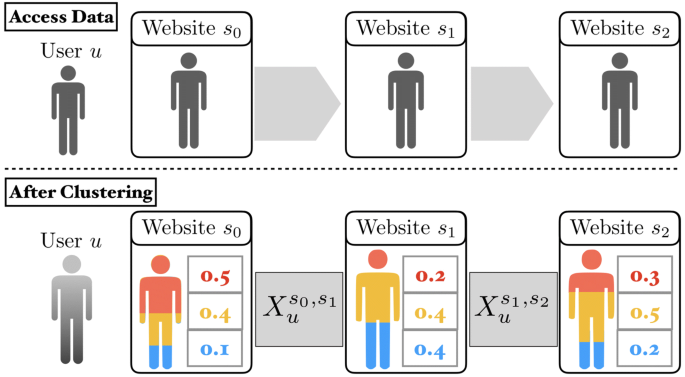Fig. 8

(A) When a user moves from website $$s_0$$ to website $$s_1$$, the following constraint is satisfied:

\begin{aligned} \Vert X_u^{s_0,s_1} \Vert _1 = \sum _{k_0,k_1 = 1}^{K}(X_u^{s_0,s_1})_{k_0,k_1} = 1 \ (\forall u,s_0,s_1) \end{aligned}
(5)

(B) Considering a certain transition of all users’ of a certain cluster $$\sum _{u \in U}{(X_u^{s_0,s_1})_{k_0,k_1}}$$ means that the number of users who travel from website $$s_0$$ (belonging to cluster $$k_0$$) to website $$s_1$$ (belonging to cluster $$k_1$$) has to be close to the value of $$P_{k_0,k_1}^{s_0,s_1}$$ obtained in Sect. 5.3. We reduce this gap, and it is denoted by $$\delta _1$$

\begin{aligned} \left| P_{k_0,k_1}^{s_0,s_1} - \sum _{u \in U}(X_u^{s_0,s_1})_{k_0,k_1}\right| \le \delta _1 \ (\forall k_0,k_1,s_0,s_1) \end{aligned}
(6)

(C) Similar to websites, each user has a feature vector that represents the average of the users’ interest in Fig. 9. The average of the memberships tends to be close to the feature vector; therefore, we reduce this gap (C), which is denoted by $$\delta _2$$ (shown in Fig. 9)Fig. 9
\begin{aligned} \left| \frac{1}{|S_u|}\sum _{s_0,s_1\in S^u}\sum _{k_0 = 1}^{K}{(X_u^{s_0,s_1})_{k_0,k_1}} - (\bar{\varvec{w}}_u)_{k_1} \right| \le \delta _2 \ (\forall u,k_1) \end{aligned}
(7)

(D) Each flow must satisfy flow conservation and can be written down using the following equation:

\begin{aligned} \sum _{k_0 = 1}^{K}(X_u^{s_0,s_1})_{k_0,k_1} = \sum _{k_0 = 1}^{K}(X_u^{s_1,s_2})_{k_1,k_0} \ (\forall k_1,s_0,s_1,s_2). \end{aligned}
(8)

We apply the parameters $$\delta _1$$ and $$\delta _2$$ to relax constraints (6) and (7) because they are challenging to be completely satisfied. Therefore, we first obtain a reasonable flow for each user by minimizing the summation of gaps.

\begin{aligned} \underset{\delta _1,\delta _2}{\mathrm{Minimize}}&\quad \delta _1 + \delta _2 \nonumber \\ {\mathrm{subject to}}&\quad {\mathrm{(A), (B), (C) \ and \ (D)}} \\&\left( X_u^{s_0,s_1}\right) _{k_0,k_1} \ge 0 \ (\forall u,s_0,s_1,k_0,k_1) \end{aligned}

#### Obtaining the Flow with Satisfying Users’ Interests

Next, we solve a similar optimization problem to obtain each user’s membership for each website, which maximizes user satisfaction. $$\delta _1$$ and $$\delta _2$$, which are the outputs of the last optimization problem, are regarded as constant values in this part. If a user stays on a website, then a dwell vector exists. How the website satisfies the user’s interest can be calculated using the inner product of the dwell vector and the user’s feature vector $$\bar{\varvec{w}}_u$$ as follows:

\begin{aligned} SF(u,s_0,s_1)&:= \sum _{k_1 = 1}^{K}\left( \sum _{k_0 = 1}^{K}{(X_u^{s_0,s_1})_{k_0,k_1}}\right) (\bar{\varvec{w}}_u)_{k_1}\\&= \sum _{k_1 = 1}^{K} x_{{u}_{k_1}}^{s_1}(\bar{\varvec{w}}_u)_{k_1}\\&= \varvec{x}_{u}^{s_1} \cdot \bar{\varvec{w}}_u. \end{aligned}

Given that it is reasonable to assume that users browse the Internet to satisfy their interests as much as possible, we set the total sum of satisfaction for all users on all websites and maximize it. Note that in this problem, we considered $$\delta _1$$ and $$\delta _2$$ as constant values.

\begin{aligned} \underset{X}{\mathrm{Maximize}}&\sum _{u \in U}\sum _{s_0,s_1 \in S_u}SF(u,s_0,s_1) \\ {\mathrm{subject \ to}}&\ {\mathrm{(A), (B), (C) \ and \ (D)}} \\&\left( X_u^{s_0,s_1}\right) _{k_0,k_1} \ge 0 \ (\forall u,s_0,s_1,k_0,k_1). \\ \end{aligned}

By solving this problem, we finally obtain the users’ membership for each website as a network flow. Each user’s network flow represents how the interest of the user’s changed during browsing.

## Obtaining the Features of a Cluster by Fitting with Weibull Distribution

By applying the proposed clustering algorithm, we obtained the memberships, which represents a user’s interests, all of which are continuous values. Based on the membership value, we fit the distribution of user’s dwell time on the webpages to the Weibull distribution and obtained the feature of each cluster from the shape parameter m of each distribution.Fig. 10

We first decomposed a user’s dwell time on each webpage corresponding to the membership (shown in Fig. 10). For example, if a user stays for t seconds on a webpage, and the user’s membership value is 0.5 for cluster 1, 0.2 for cluster 2, and 0.3 for cluster 3, the histogram for cluster 1 is added by 0.5 people dwelling t seconds. This is also performed for cluster 2 and cluster 3. In this way, we prepare K histograms for fitting with the Weibull distribution, as illustrated in Fig. 11. We fit a single Weibull distribution per webpage s per cluster k using the EM algorithm, and the probability density function is denoted by $$f^s_k$$. Recall $$N^s$$ is the number of users visiting a webpage s and $$N_k^s$$ is the summation of the rate of memberships belonging to the cluster k over users visiting the webpage s, as mentioned in Sect. 4. We obtained the mixture Weibull density function of the webpage s$$F_s(x)$$ by using the following equation:

\begin{aligned} F_s(x) := \sum _{k=1}^K f^s_k(x)\frac{N^s_k}{N^s}. \end{aligned}

By applying $$F_s(x)$$, we can check how our proposed clustering method is better by calculating the Kullback–Leibler divergence (shown in Sect. 3) from other clustering methods. Finally, we calculate the proposed index “Attractiveness Factor” (defined in Sect. 4) and evaluate each website’s attractiveness. Numerical Experiments are shown in the next section.Fig. 11

## Experiment

In this section, we describe the numerical experiments that were conducted by solving extremely large-scale optimization problems generated by the real access data of Yahoo Japan News. We conducted two experiments to demonstrate the advantage of our clustering algorithm and to evaluate the potential of AF by comparing it with other indices.

### Experiment Environment

• Dataset: We utilized the real access data of the Yahoo Japan News from Yahoo Japan Corporation. We selected three 1-h access datasets, 8:00–9:00 a.m., 2:00–3:00 p.m., and 8:00–9:00 p.m. on July 1, 2018, each of which contains about two million access data, because we want to check how time influences indices. In addition, we picked up three 1-h access dataset, 8:00–9:00 a.m. and 8:00–9:00 p.m. on April 1, 2019, and 8:00–9:00 a.m. and 2:00–3:00 p.m. on February 28, 2019, to check the ability of Attractiveness Factor.

• Software: We conducted numerical experiments with Python 3.6.3 and utilized Nimfa and Gurobi optimizer 8.0.1 for applying nonnegative matrix factorization and solving the linear optimization problem, respectively.

• Platforms: The specifications of our experimental platforms are provided in Table 4.

### Validation of Clustering

We first describe the advantage of our clustering algorithm. We performed clustering using many clustering methods and generated mixture Weibull distributions, as mentioned in Sects. 5 and 6. We calculate the Kullback–Leibler divergence between each mixture Weibull distribution based on the clustering and EM algorithm. By calculating the weighted relative error of them with considering the number of website visitors, we compare the clustering algorithms.

#### Evaluation Metric

The error metric is defined as follows:

\begin{aligned}&\text{Loss} = \sum _{s \in S} \frac{{\text{KL}}(p_s||q^{Cl}_s)-{\text{KL}}(p_s||q^{{\text{MLE}}}_s)}{{\text{KL}}(p_s||q^{{\text{MLE}}}_s)} \chi _\alpha (N_s) \\&\chi _\alpha (x) = {\left\{ \begin{array}{ll} \log (x) \ &{} (x \ge \alpha ) \\ 0 \ &{} ({\mathrm{otherwise}}) \end{array}\right. } \end{aligned}
• $$p_s$$: Probability density function of users’ dwell time on website s

• $$q^{Cl}_s$$: Probability density function of mixture Weibull distribution with clustering method

• $$q^{MLE}_s$$: Probability density function of mixture Weibull distribution with maximum likelihood method with utilizing EM algorithm (100 iterations) 

• $$N_s$$: Number of users visiting website s

• $$\alpha$$: Parameter of lower bound

We describe the advantage of our approach by comparing our clustering algorithm with other clustering methods. For each clustering method, its mixture Weibull distribution is calculated by employing our method mentioned in Sects. 5 and 6. We then calculate the Kullback–Leibler divergence (KL divergence) from the dwell time distribution to each mixture Weibull distribution. In addition, we obtain a mixture Weibull distribution calculated with the maximum likelihood method. We then calculate KL divergence from the dwell time distribution to the mixture Weibull distribution, and we regard the divergence as the baseline. Since the KL divergence measures the difference between two probability distributions, we combine the two KL divergences and precisely evaluate each clustering approach based on the loss. In the experiment, we apply the EM algorithm as the maximum likelihood method, which is one of the approximation methods. We ran the EM algorithm with at most 100 iterations because of the computation time constraints. We also set the parameter $$\alpha$$ to avoid poor Weibull distribution fitting when the number of visitors is tiny.

#### Compared Methods

We compared our clustering method with other clustering methods. We performed clustering using various clustering methods and obtained a mixture Weibull distribution by mixing the single Weibull distributions for each cluster. The compared methods include:

• Fuzzy c-means  : Group behavior matrix into overlapping clusters using the fuzzy c-means algorithm, which is a typical fuzzy clustering method.

• NMF : Feature vector per person ($$\bar{\varvec{w}}_u$$), which is the output of the NMF, is just applied to the dwell vector.

#### Results

The results of calculating the Loss with respect to $$\alpha = 1000$$ are shown in Table 5.

Table 5 shows that our algorithm performed better than the other algorithms in all the datasets. Compared to other clustering methods, our clustering method enabled us to obtain the mixture Weibull distribution which was the closest to the mixture Weibull distribution acquired by EM algorithm. Figure 12 shows some of the histograms of the users’ dwell time distribution and the mixture Weibull distribution generated by each clustering method. The mixture Weibull distribution based on our clustering method is capable of expressing the complexity of dwell time distribution. We performed other clustering methods such as the CARD algorithm, but it remains incomplete as the memory gets exhausted. Therefore, such an algorithm is not appropriate for extremely large-scale datasets.Fig. 12

### Evaluation of Attractiveness Factor

Here, we focus on the ability of AF by comparing it with other indices. We performed experiments using the three datasets mentioned above. The baselines include:

• Number of access (Num)

• Average dwell time on the website (Ave)

• Exit rate (Ex) (The rate of users who quit browsing from the website)

In addition, we show the scatter diagrams in Figs. 13, 14, and 15, which show the relationship between AF and number of visitor, average dwell time, and exit rate, respectively. A website is plotted on three different scatter plots. The first rows in Figs. 13, 14, and 15 are the scatter diagrams between AF and the number of visitors, the average dwell time on the website, and exit rate from top to bottom. Figures 13, 14, and 15 show the scatter diagrams made by the access log of July 1, 2018, April 1, 2019, and February 28, 2019, respectively. We show the correlation coefficient r in the title. These figures imply the following features of AF.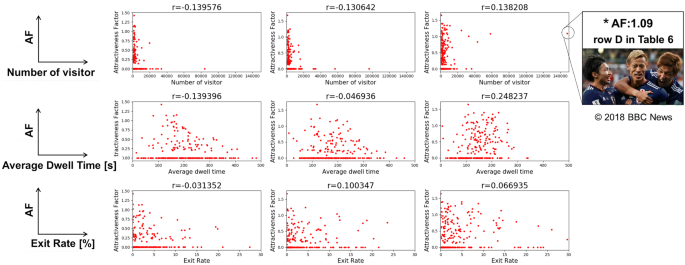Fig. 13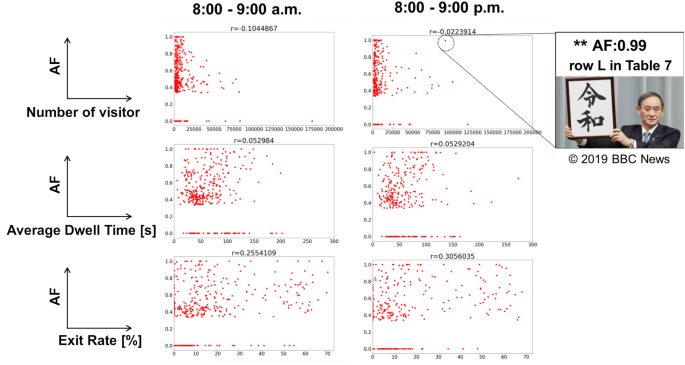Fig. 14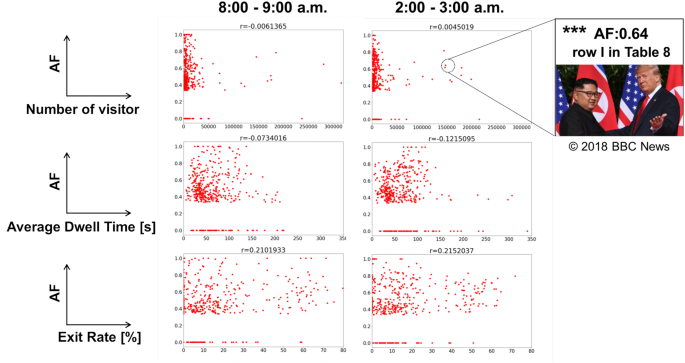Fig. 15
• Many users visited the category pages. We assume that the category pages were used as a via point and users left early from these webpages. Therefore, the category pages were assumed to be evaluated zero by AF, which matches our inspections.

• AF rates high for the webpages which retain peoples’ interests regardless of the number of visitors in all datasets. Examples are shown below:

• $$^{*}$$News about Soccer World Cup (Fig. 13)

• $$^{**}$$News about Japan’s new imperial era “REIWA” (Fig. 14)

• $$^{***}$$News about the meeting between North Korean Supreme Leader Kim Jong-un and US President Donald Trump (Fig. 15)

• There is no correlation between AF and three well-used indices, which is guaranteed by calculating the correlation coefficient. It implies that AF can observe from the point of view different from them.

These results ensure that AF is an appropriate and suitable index to evaluate the websites’ attractiveness.

We show the result of several websites in Tables 6, 7 and 8. We picked up several websites per category, which are shown in one Table.

AF and well-used indices of one website are shown in one column in each table. If we only focus on the number of visitors, category pages are rated high. It implies the following features of AF.

• Row F in Table 6 was the news about the Soccer World Cup. Among the three datasets, the average dwell time and exit rate did not change dramatically. However, we can observe the difference of AF among the three datasets. The observation indicates that AF can extract the feature which cannot be distinguished with well-used indices. The soccer fans seemed to access the webpages about soccer before the game at midnight.

• Rows F, L in Table 7 and rows D, I, L in Table 8 are rated highly by AF. Most of the visitors seemed to be satisfied with the webpage.

• On all category pages of Tables 6, 7 and 8, there were many visitors with low exit rates, which means that the webpages were used as the via points. We consider that many users left the webpage early. It causes that the shape parameter m of fitted Weibull distribution is less than one so that AF evaluates zero for the via points. AF rates zeros in all category pages shown in Tables 6, 7 and 8, which matches our inspections.

• The webpage I in Table 8 had more access than the category pages, whereas the AF valued positively. AF can evaluate the attractive webpages regardless of the number of visitors.

• Rows F and J in Table 6 have a low exit rate and a long average dwell time. These results imply that users were likely to continue browsing after enjoying the webpages. These webpages seem to have high attractiveness, and they are rated by high by AF as expected.

• News about soccer (rows D–F in Table 6) is observed high AF with time. The Japanese national soccer team would play the first round of the World Cup tournament the next day, which may have caused this result. However, the average dwell time on the website did not change dramatically.

## Conclusion

In this paper, we proposed a new framework for measuring a web page’s attractiveness. Our proposed index named “Attractiveness factor” (AF) can measure the extent to which a webpage retains the users’ attention. To the best of our knowledge, this is the first index focusing on both users’ dwell time distribution and the dynamics of users’ interests. Our framework is applied using the following three steps: First, we captured the dynamics of users’ interests during browsing by solving NMF and constrained network flow problems. It is a soft clustering method that allows users to have some types of interests. This is also the first study that obtains the transition of users’ interests according to network flow. Second, the feature of each cluster of each webpage can be obtained by fitting the dwell time distribution with Weibull distribution, which is a well-known tool for understanding object defects. Finally, by applying the results of clustering and fitting, we can obtain AF for each webpage. The potential of AF is shown through large-scale numerical experiments with real access data of Yahoo Japan News. AF rates zero for category pages; however, a large value for webpages that retain users’ attention. Moreover, we can determine the differences of users’ interests for each webpage, depending on the time, by calculating Attractiveness Factor for access data in the morning, daytime, and at night. The application of AF yielded much better results than expected, and it is expected to be a promising index for evaluating websites.

AF can play an essential role in measuring the attractiveness of contents in many situations, such as advertisements, retail, and online education. In such situations, we regard the input as any transition history instead of the web access data. We intend to apply AF for such situations in the future.

In addition, we will establish the underlying technology of a system that maximizes user entertainment and learning. In this study, we analyzed only web access data; however, we can expand this to complex data analysis for geographical information, biometric information, accompanying the text. Furthermore, we aim to establish a new recommendation system that includes the following features: (1) Online recommendation methods considering the changing condition, including internal conditions (biological information and intention) and external conditions (social situation), as well as personal profiles (interests and concerns). (2) Recommendation method for content that can draw out the potential interests of users, not simply content following the user’s current and past interests.

## References

1. 1.

Agichtein E, Brill E, Dumais S, Brill E, Dumais S (2006) Improving web search ranking by incorporating user behavior. In: Proceedings of SIGIR 2006. https://www.microsoft.com/en-us/research/publication/improving-web-search-ranking-by-incorporating-user-behavior/. Accessed 9 April 2019

2. 2.

Bar-Ilan J, Levene M (2015) The hw-rank: an h-index variant for ranking web pages. Scientometrics 102(3):2247–2253. https://doi.org/10.1007/s11192-014-1477-2

3. 3.

Barbieri N, Silvestri F, Lalmas M (2016) Improving post-click user engagement on native ads via survival analysis. In: Proceedings of the 25th international conference on World Wide Web, WWW ’16, pp 761–770. International World Wide Web Conferences Steering Committee, Republic and Canton of Geneva, Switzerland. https://doi.org/10.1145/2872427.2883092

4. 4.

Bhavithra J, Saradha A (2018) Personalized web page recommendation using case-based clustering and weighted association rule mining. Clust Comput. https://doi.org/10.1007/s10586-018-2053-y

5. 5.

Brunet JP, Tamayo P, Golub TR, Mesirov JP (2004) Metagenes and molecular pattern discovery using matrix factorization. Proc Natl Acad Sci 101(12):4164–4169

6. 6.

Budylin R, Drutsa A, Katsevm I, Tsoy V (2018) Consistent transformation of ratio metrics for efficient online controlled experiments. In: The eleventh ACM international conference on web search and data mining, WSDM ’18. ACM, New York. https://doi.org/10.1145/3159652.3159699

7. 7.

Castellano G, Mesto F, Minunno M, Torsello MA (2007) Web user profiling using fuzzy clustering. In: Proceedings of the 7th international workshop on fuzzy logic and applications: applications of fuzzy sets theory, WILF ’07. Springer, Berlin, , pp 94–101. https://doi.org/10.1007/978-3-540-73400-0_12

8. 8.

Duong VH, Lee YS, Ding JJ, Pham BT, Bui MQ, Wang JC et al (2018) Projective complex matrix factorization for facial expression recognition. EURASIP J Adv Signal Process 2018(1):10

9. 9.

Elmahdy EE, Aboutahoun AW (2013) A new approach for parameter estimation of finite Weibull mixture distributions for reliability modeling. Appl Math Model 37(4):1800–1810

10. 10.

Gopalakrishnan T, Sengottvelan P (2014) Discovering user profiles for web personalization using em with Bayesian classification. Aust J Basic Appl Sci 8(3):53–60

11. 11.

Kathuria A, Jansen BJ, Hafernik C, Spink A (2010) Classifying the user intent of web queries using k-means clustering. Internet Res 20(5):563–581

12. 12.

Khusumanegara P, Mafrur R, Choi D (2015) Profiler for smartphone users interests using modified hierarchical agglomerative clustering algorithm based on browsing history. In: Khalil I, Neuhold E, Tjoa AM, Xu LD, You I (eds) Information and communication technology. Springer, Cham, pp 89–96

13. 13.

Kim Y, Hassan A, White R.W, Zitouni I (2014) Modeling dwell time to predict click-level satisfaction. In: Proceedings of the 7th ACM international conference on web search and data mining, WSDM ’14. ACM, New York, pp 193–202. https://doi.org/10.1145/2556195.2556220

14. 14.

Kleinberg JM (1999) Authoritative sources in a hyperlinked environment. J ACM 46(5):604–632. https://doi.org/10.1145/324133.324140

15. 15.

Lagun D, Lalmas M (2016) Understanding user attention and engagement in online news reading. In: Proceedings of the ninth ACM international conference on web search and data mining, WSDM ’16. ACM, New York, pp 113–122. https://doi.org/10.1145/2835776.2835833

16. 16.

Lee DD, Seung HS (2000) Algorithms for non-negative matrix factorization. In: Proceedings of the 13th international conference on neural information processing systems, NIPS’00. MIT Press, Cambridge, pp 535–541. http://dl.acm.org/citation.cfm?id=3008751.3008829

17. 17.

Lim W, Du R, Park H (2018) Codinmf: co-clustering of directed graphs via NMF. In: Proceedings of the 32nd AAAI conference on artificial intelligence

18. 18.

Liu C, White RW, Dumais S (2010) Understanding web browsing behaviors through Weibull analysis of dwell time. In: Proceedings of the 33rd international ACM SIGIR conference on research and development in information retrieval, SIGIR ’10. ACM, New York, pp 379–386. https://doi.org/10.1145/1835449.1835513

19. 19.

Liu Y, Gao B, Liu TY, Zhang Y, Ma Z, He S, Li H (2008) Browserank: letting web users vote for page importance. In: Proceedings of the 31st annual international ACM SIGIR conference on research and development in information retrieval, SIGIR ’08. ACM, New York, pp 451–458. https://doi.org/10.1145/1390334.1390412

20. 20.

Lu H, Zhang M, Ma S (2018) Between clicks and satisfaction: study on multi-phase user preferences and satisfaction for online news reading. In: The 41st international ACM SIGIR conference on research and development in information retrieval, SIGIR ’18. ACM, New York, pp 435–444. https://doi.org/10.1145/3209978.3210007

21. 21.

Bhuvaneswari MS, Muneeswaran K, Sakthi Priya KS (2018) Fuzzy clustering of augmented web user sessions. Int J Pure Appl Math 118(20):1153–1161

22. 22.

Nasraoui O, Frigui H, Krishnapuram R, Joshi A (2000) Extracting web user profiles using relational competitive fuzzy clustering. Int J Artif Intell Tools 09(04):509–526. https://doi.org/10.1142/S021821300000032X

23. 23.

Nikolaev K, Drutsa A, Gladkikh E, Ulianov A, Gusev G, Serdyukov P (2015) Extreme states distribution decomposition method for search engine online evaluation. In: Proceedings of the 21th ACM SIGKDD international conference on knowledge discovery and data mining, KDD ’15. ACM, New York, pp 845–854

24. 24.

Page L, Brin S, Motwani R, Winograd T (1999) The pagerank citation ranking: bringing order to the web. Technical report, Stanford InfoLab

25. 25.

Poornalatha G, Raghavendra PS (2011) Web user session clustering using modified k-means algorithm. In: Abraham A, Lloret Mauri J, Buford JF, Suzuki J, Thampi SM (eds) Advances in computing and communications. Springer, Berlin, pp 243–252

26. 26.

Sculley D, Malkin RG, Basu S, Bayardo RJ (2009) Predicting bounce rates in sponsored search advertisements. In: Proceedings of the 15th ACM SIGKDD international conference on knowledge discovery and data mining, KDD ’09. ACM, New York, pp 1325–1334. https://doi.org/10.1145/1557019.1557161

27. 27.

Vasiloudis T, Vahabi H, Kravitz R, Rashkov V (2017) Predicting session length in media streaming. In: Proceedings of the 40th international ACM SIGIR conference on research and development in information retrieval, SIGIR ’17. ACM, New York, pp 977–980. https://doi.org/10.1145/3077136.3080695

28. 28.

Wang C, Kalra A, Borcea C, Chen Y (2016) Webpage depth-level dwell time prediction. In: Proceedings of the 25th ACM international on conference on information and knowledge management, CIKM ’16. ACM, New York, pp 1937–1940. https://doi.org/10.1145/2983323.2983878

29. 29.

Zhou C, Bai J, Song J, Liu X, Zhao Z, Chen X, Gao J (2017) Atrank: an attention-based user behavior modeling framework for recommendation. CoRR arXiv:1711.06632

Download references

## Acknowledgements

This research project was supported by the Japan Science and Technology Agency (JST), the Core Research of Evolutionary Science and Technology (CREST), the Center of Innovation Science and Technology based Radical Innovation and Entrepreneurship Program (COI Program), JSPS KAKENHI Grant No. JP 16H01707.

## Author information

Authors

### Corresponding author

Correspondence to Akihiro Yoshida.

## Rights and permissions

Open Access This article is distributed under the terms of the Creative Commons Attribution 4.0 International License (http://creativecommons.org/licenses/by/4.0/), which permits unrestricted use, distribution, and reproduction in any medium, provided you give appropriate credit to the original author(s) and the source, provide a link to the Creative Commons license, and indicate if changes were made.

Reprints and Permissions